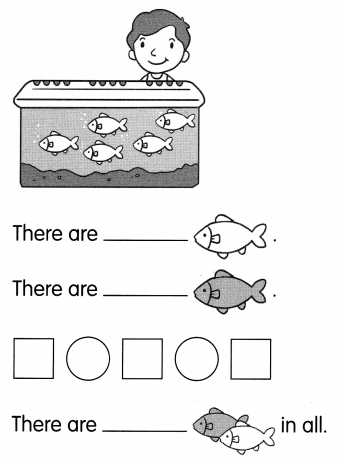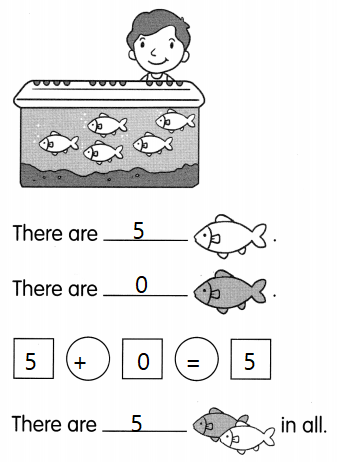This handy Math in Focus Grade 1 Workbook Answer Key Chapter 3 Practice 3 Making Addition Stories detailed solutions for the textbook questions.

Use the pictures to make addition stories.

Example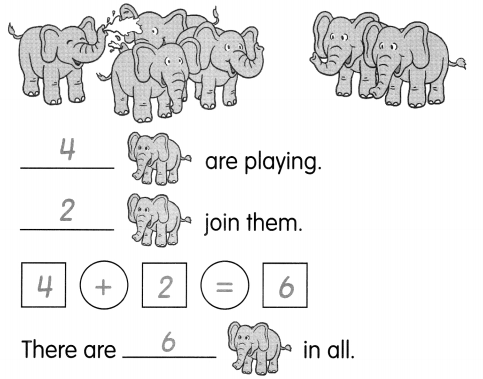Question 1.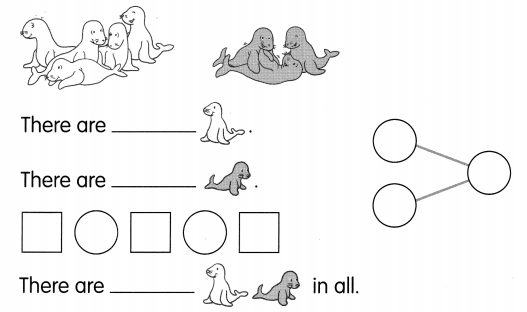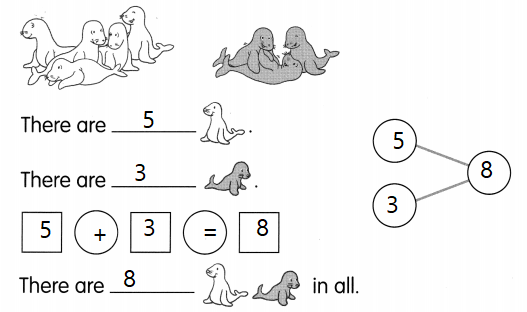Explanation:
There are 5 white dolphins and 3 colored dolphins
5 + 3 = 8
The sum of 5 and 3 is 8

Question 2.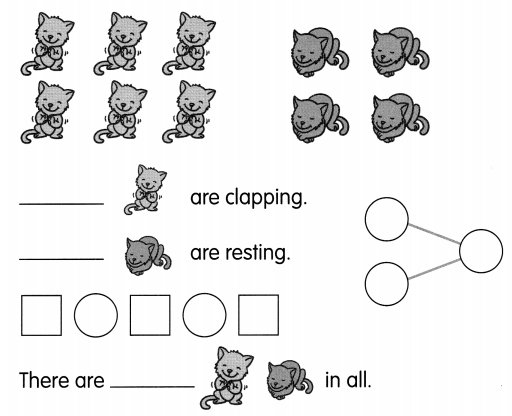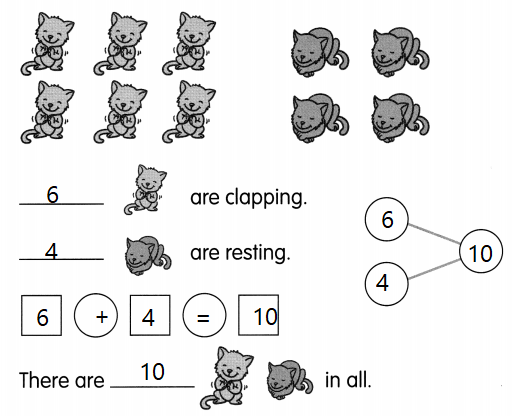Explanation:
cats are clapping and 4 are sleeping
6 + 4 = 10
The sum of 6 and 4 makes 10

Question 3.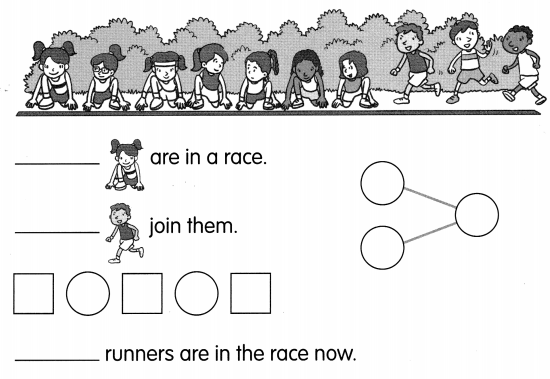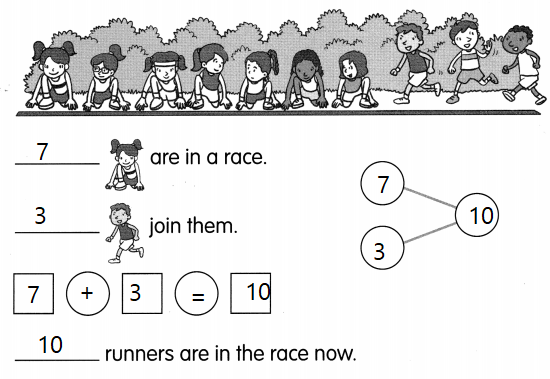Explanation:
there are 7 girls in the competition
and 3 boys joined them
7 + 3 = 10
The sum of 7 and 3 is 10.

Question 4.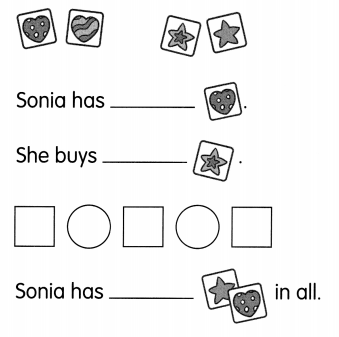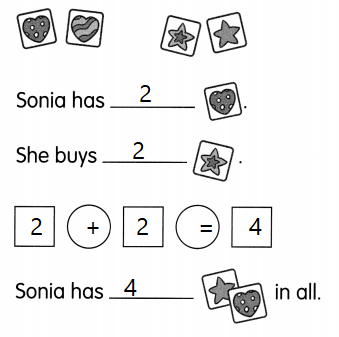Explanation:
Sonia has 2 star stickers
and 2 heart stickers
2 + 2 = 4
The sum of 2 and 2 is 4

Question 5.